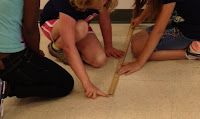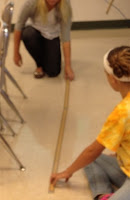### How Many Decimeters?How many decimeters is it from the front of the room to the back of the room? This is the question I posed to my students this week.Our team is currently working on an interdisciplinary unit, and one part of it includes metric conversions. The students are learning about the metric system  in science, so I'm supporting that in math class. To being this particular lesson, students brainstormed any possible units that could be used to measure from the front of the room to the back, and they brainstormed tools that could be used to do the measuring. After some discussion about the difference between yardsticks and meter sticks, I gave the students the task of measuring that distance in decimeters. So, they set about the task. They knew what a meter was, and seemed to know where the centimeters were on the meter stick, but they didn't know exactly what a decimeter was, and I wouldn't tell them:-)    I wanted them to struggle some, and try to figure it out on their own. When they were finished measuring, they wrote their measurements on the board.It was interesting to note the different measuring methods and the results of this activity in my 4 different classes. Some groups used 2 meter sticks and alternated the one they moved, to help measure more accurately. Others used just one and used a pencil or their finger to mark where one meter stick ended and the next should begin.

Results: All of the groups in my first class measured in centimeters, so we took the time to review where the centimeters were located on the meter stick. After discussion, we arrived at the conclusion that the decimeters were the multiples of 10 on the meter stick, and the students then remeasured the distance, to arrive at the correct answer. The fabulous thing about this class is that it has only 14 students, so it allows me to spend more time listening and talking to every student as they work through the problem.The second class was much like the first, but the 3rd math class had one group that correctly figured out the decimeter measurement.    The fourth class, also a small class, really impressed me. Many of these students asked if they could use a resource to help them figure out what a decimeter was. Some used their science journal to check notes they had written; some used their math books! (I do have a poster in my room with the prefix "steps," but I don't think any of them looked at it...)   All of the groups in this class found reasonable decimeter answers for the measurement (except for one....I think they just counted the number of meter sticks incorrectly, because I could hear them correctly explaining to each other how they arrived at the number of decimeters). I was so impressed with this group!

### Memory Wheels - First Day, Last Day, and Any Day in Between!

This post has been moved to:  http://www.cognitivecardiowithmsmm.com/blog/memory-wheels-first-day-last-day-and-any-day-in-between

### Math Class - First Day Activity

Rectangle of pentominoes Many 6th graders seem to have a pretty negative attitude about math, so I try to do something interesting to "grab" them during our first class. Last year, during the first math class, we spent part of the period working with pentominoes. Before working with the pentominoes, however, we played a name game so we could learn each others' names (I find it impossible to start anything else if I don't know some names, and fortunately, I learn them fairly quickly). rectangle outline For the activity, I divided the students into groups of 3 or 4. The directions for the activity were not complicated - the task was to make a rectangle, using all of the pentominoes. I gave students an outline of the rectangle, as pictured to the left, so they would know the correct size of the rectangle. The squares in the grid are each one inch. The rectangle is 5 squares (inches) wide and 13 inches long (13 inches includes the row that has the "Pent

### How Much Math Homework??

I am very curious about math homework in middle school, from a teacher perspective:     How much math homework do you give?     What kind of homework do you give?     How do you go over it in class? Let me explain why I ask these questions. I have taught 6th grade math for eight years, and every year, my goal is to "perfect" the homework issue. My basic issue is that I feel that I spend too much time going over it (not necessarily every day, but often). In the past, we have reviewed homework in the following ways:    1. going over answers as a class    2. self-checking answers that are on the board and sharing any questions    3. partner-checking and then verifying    4. choosing only a few problems to check When I taught elementary school (for 12 years), I never seemed to have this problem....we had 60 minutes for class and I never struggled to fit everything in. But at middle school, we have 44 minutes (minus time to switch for classes), and I just haven't fo